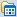# 大悟还俗

posts - 236, comments - 8, trackbacks - 0, articles - 0
新随笔 :: 联系 :: 订阅:: 管理### 公告

A D O以异步的方式来处理数据。

1) 在D e l p h i集成开发环境中关闭所有的项目。
2) 建立一个新的应用程序项目，在主窗体中放入TA D O C o n n e c t i o n对象连接到

3) 在主窗体中放入TA D O D a t a S e t组件。设定它的C o n n e c t i o n属性值为步骤2) 的
TA D O C o n n e c t i o n，并且在它的C o m m a n d Te x t属性值中使用Select * from ADOTe s t D a t a s

5) 放入T D B N a v i g a t o r和T D B G r i d组件，设定它们的D a t a S o u r c e组件为步骤4加

6) 在主窗体中放入两个T B u t t o n组件以及一个T P r o g r e s s B a r组件，并且设定它

7) 最后在主窗体中放入一个TA D O C o m m a n d组件。设定它的C o n n e c t i o n属性

count(*) from ADOTe s t D a t a s以便从A D O Te s t D a t a s数据表中取得所有数据的笔数。
8) 在主窗体的O n A c t i v a t e事件处理程序中撰写如下的程序代码：

p r o c e d u r e TForm1.FormActivate(Sender: TObject);
v a r
sRecNo : String;
b e g i n
sRecNo := IntToStr(ProgressBar1.Max);
Self.Caption := '共有' + sRecNo + ' 笔数据' ;
e n d ;
O n A c t i v a t e事件处理程序首先执行TA D O C m m a n d的S Q L命令，并且从它回传

9) 双击窗体中的e o A s y n c F e t c h N o n B l o c k i n g按钮，并且在它的O n C l i c k事件处

p r o c e d u r e TForm1.Button2Click(Sender: TObject);
b e g i n
t r y
f i n a l l y
lStart := GetTickCount;
e n d ;
e n d ;

A D O Te s t D a t a s数据表中取得数据。
10) 双击窗体中的e o A s y n c F e t c h按钮，并且在它的O n C l i c k事件处理程序中撰

p r o c e d u r e TForm1.Button1Click(Sender: TObject);
b e g i n
t r y
f i n a l l y
lStart := GetTickCount;
1 1 2 Delphi 5.x ADO/MTS/COM+高级程序设计篇

e n d ;
e n d ;

A D O Te s t D a t a s数据表中取得数据。

11) 在步骤3) 的TA D O D a t a S e t组件的O n F e t c h P r o g r e s s事件处理程序中撰写如下

Progress, MaxProgress: Integer; v a r EventStatus: TEventStatus);
b e g i n
ProgressBar1.Position := Progress;
e n d ;

12) 在步骤3) 的TA D O D a t a S e t组件的O n F e t c h C o m p l e t e事件处理程序中撰写如

c o n s t Error: Error; v a r EventStatus: TEventStatus);
b e g i n
lEnd := GetTickCount;
S h o w M e s s a g e ( '总共花了' + FloatToStr((lEnd - lStart) / 1000.0) + ' 秒' ) ;
e n d ;

A D O Te s t D a t a s这个范例数据表中数据的画面。从图中可以看到，当A D O存取数据

ExecuteOptions
eoAsyncExecute 异步执行
eoAsyncFetch  异步提取
eoAsyncFetchNonBlocking 无阻塞方式异步提取
eoExecuteNoRecords 无返回记录执行